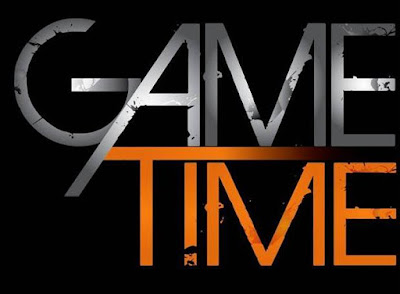Answer this question and be sure you have your free airtime or data to any network you desire.

See below :Game Time!! Answer This Question And Win Free Airtime And DataReviewed by InoutNaija Staff on 16:41:00 Rating: 5

1.2 *1 + 3 *2 = 2+6 = 8

3 *2 + 7 *3 = 6+21 =27

4 *3 + 5 *4 = 12+20 = 32

5 *4 + 8 *5 = 20+40 = 60

6 *5 + 7 *6 = 30+42 = 72

7 *6 + 8 *7 = 42+56 = 98 hence , 7+8 = 98

2.2*1+3*2=2+6=8
3*2+7*3=6+21=27
4*3+5*4=12+20=32
5*4+8*5=20+40=60
6*5+7*6=30+42=72
7*6+8*7=42+56=98, therefore 7+8=98

3.2*1+3*2=2+6=8
3*2+7*3=6+21
4*3+5*4=12+20=32
5*4+8*5=20+40=60
6*5+7*6=30+42=72
7*6+8*7=42+56=98
7+8===98

4.mtcheewwww i left this blog long ago because of this woman "WEREY" behaviour.. wickedness is her middle name.. quarrelling with all her friends everywhere up and down.. Go and marry na and see what will happen...hahahahahha.. Your time will soon be up!

5.Thanks I got the airtime.

6.Nice one

7.8.9.10.Take advantage of our blog traffic and promote your product to millions of viewers.

For advert placements and other Enquiries, kindly send us a mail at [email protected] or call 08025707646, 07031338542

Visit the Play stores to Download our mobile app and stay up -to-date.